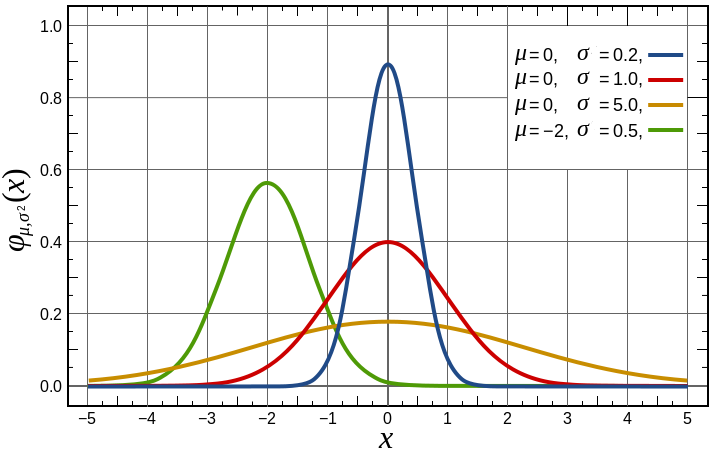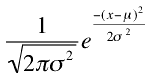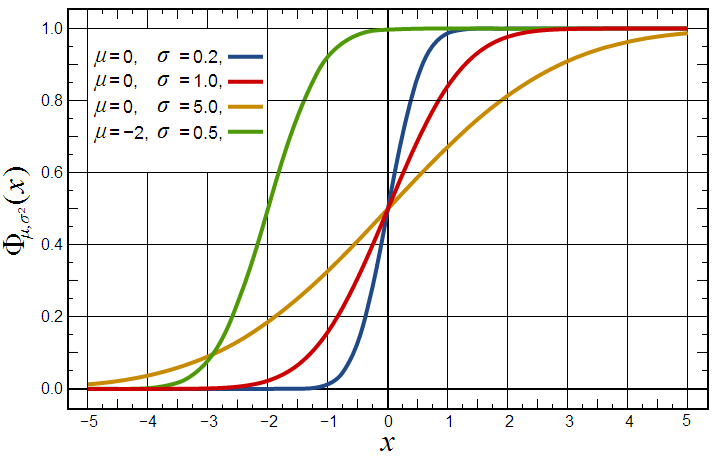# Statistics for Decision Makers - 07.01 - Normal Distributions

Title

07.01 - Normal Distributions
Author
Bernard Szlachta (NobleProg Ltd) bs@nobleprog.co.uk
Footer
www.NobleProg.co.uk
Subfooter

Training Courses Worldwide

## Normal Distributions。

• Most of the statistical analyses presented are based on the bell-shaped or normal distribution
• We learn how to calculate probabilities based on the normal distribution
• A frequently used normal distribution is called the Standard Normal distribution (AKA Gauss Distribution)
• The binomial distribution can be approximated by a normal distribution
• A binomial distribution is used for discrete variables
• A normal distribution is for continuous variables

# Bell Curve。

• "Gaussian curve" (μ = 0, σ = 1)
• Although Gauss played an important role in its history, de Movire first discovered the normal distribution
• There is no single "the normal distribution" since there are many normal distributions which differ in their means and standard deviations
• Normal distributions can differ in their means and in their standard deviations
• The distributions below, as well as all other normal distributions, are symmetric with relatively more values at the centre of the distribution and relatively few in the tails
```=NORMDIST(1,0,1,FALSE) = 0.2419707245
```# The density of the normal distribution。• The density of the normal distribution (the height for a given value on the x axis) is shown above
• The parameters μ and σ are the mean and standard deviation, respectively, and define the normal distribution
• The symbol e is the base of the natural logarithm and π is the constant pi
• Since this is a non-mathematical treatment of statistics, do not worry if this expression confuses you

## Normal Cumulative Distribution。```=NORMDIST(1,0,1,true) = 0.8413447461
```

# Features of Normal Distributions。

1. Normal distributions are symmetric around their mean
2. The mean, median, and mode of a normal distribution are equal
3. The area under the normal curve is equal to 1.0
4. Normal distributions are denser in the center and less dense in the tails
5. Normal distributions are defined by two parameters, the mean (μ) and the standard deviation (σ)
6. 68% of the area of a normal distribution is within one standard deviation of the mean
7. Approximately 95% of the area of a normal distribution is within two standard deviations of the mean

# Quiz

1

Which are other names for the normal distribution? Select all that apply.

 Typical curve Gaussian curve Regular distribution Galileo curve Bell-shaped curve Laplace's distribution

Gaussian curve, Bell-shaped curve

2

Select all of the statements that are true about normal distributions.

 A:They are symmetric around their mean B:The mean, median, and mode are equal C:They are defined by their mean and skew D:The area under the normal curve is equal to 1.0 E:They have high density in their tails F:They are discrete distributions